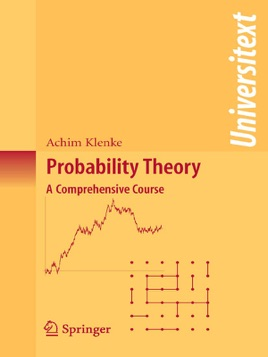• \$69.99

## Publisher Description

Probabilistic concepts play an increasingly important role in mathematics, physics, biology, financial engineering and computer science. They help us to understand magnetism, amorphous media, genetic diversity and the perils of random developments on the financial markets, and they guide us in constructing more efficient algorithms.

This text is a comprehensive course in modern probability theory and its measure-theoretical foundations. Aimed primarily at graduate students and researchers, the book covers a wide variety of topics, many of which are not usually found in introductory textbooks, such as:

limit theorems for sums of random variables;

martingales;

percolation;

Markov chains and electrical networks;

construction of stochastic processes;

Poisson point processes and infinite divisibility;

large deviation principles and statistical physics;

Brownian motion; and

stochastic integral and stochastic differential equations.

The theory is developed rigorously and in a self-contained way, with the chapters on measure theory interlaced with the probabilistic chapters in order to display the power of the abstract concepts in the world of probability theory. In addition, plenty of figures, computer simulations, biographic details of key mathematicians, and a wealth of examples support and enliven the presentation.